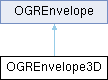GDAL
OGREnvelope3D Class Reference

Simple container for a bounding region in 3D. More...

`#include <ogr_core.h>`

Inheritance diagram for OGREnvelope3D:## Public Member Functions

OGREnvelope3D ()
Default constructor. More...

OGREnvelope3D (const OGREnvelope3D &oOther)
Copy constructor.

OGREnvelope3Doperator= (const OGREnvelope3D &)=default
Assignment operator.

bool Is3D () const
Returns TRUE if MinZ and MaxZ are both valid numbers.

int IsInit () const
Return whether the object has been initialized, that is, is non empty.

void Merge (OGREnvelope3D const &sOther)
Update the current object by computing its union with the other rectangle.

void Merge (OGREnvelope const &sOther)
Update the current object by computing its union with the other rectangle.

void Merge (double dfX, double dfY, double dfZ)
Update the current object by computing its union with the provided point.

void Intersect (OGREnvelope3D const &sOther)
Update the current object by computing its intersection with the other rectangle.

int Intersects (OGREnvelope3D const &other) const
Return whether the current object intersects with the other rectangle.

int Contains (OGREnvelope3D const &other) const
Return whether the current object contains the other rectangle.

void Merge (double dfX, double dfY)
Update the current object by computing its union with the provided point.

void Intersect (OGREnvelope const &sOther)
Update the current object by computing its intersection with the other rectangle.

int Intersects (OGREnvelope const &other) const
Return whether the current object intersects with the other rectangle.

int Contains (OGREnvelope const &other) const
Return whether the current object contains the other rectangle.

bool operator== (const OGREnvelope &other) const
Return whether the current rectangle is equal to the other rectangle.

bool operator!= (const OGREnvelope &other) const
Return whether the current rectangle is not equal to the other rectangle.

## Public Attributes

double MinZ
Minimum Z value.

double MaxZ
Maximum Z value.

double MinX
Minimum X value.

double MaxX
Maximum X value.

double MinY
Minimum Y value.

double MaxY
Maximum Y value.

## Detailed Description

Simple container for a bounding region in 3D.

## ◆ OGREnvelope3D()

 OGREnvelope3D::OGREnvelope3D ( )
inline

Default constructor.

Defines an empty rectangle

The documentation for this class was generated from the following file: